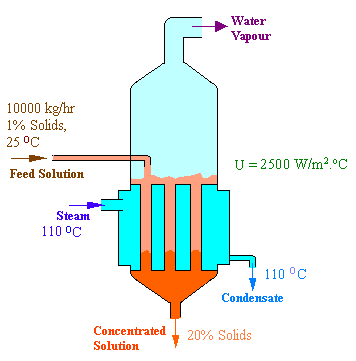### Steam Requirement of Single Effect Evaporator

10,000 kg/hr of an aqueous feed containing 1% dissolved solids is to be concentrated to 20% solids, in a single effect evaporator. The feed enters at 25oC. The steam chest is fed with saturated steam at 110oC. The absolute pressure maintained in the evaporator is such that the water will boil at 55oC. The boiling point elevations are as follows:

Feed: 0.2oC
20% solution: 15oC

The overall heat transfer coefficient, under normal operating conditions would be 2500 W/m2.oC

Estimate the steam requirement assuming no sub cooling of condensate, heat load on the condenser, and the heat transfer area.

Calculations: The given data are represented in the following diagram.Let us have the following notations:

Feed: F

Concentrated product: P

Water vapor: V

Steam: S

Mass balance:

Solid balance:

F x 0.01 = P x 0.2

P = 10000 x 0.01 / 0.2 = 500 kg/hr

V = F - P = 10000 - 500 = 9500 kg/hr

Energy balance:

Temperature of Water vapor, leaving from the evaporator

= 55oC + Boiling point elevation = 55oC + 15oC = 70oC

Enthalpy of feed at 25oC (HF)= 104.8 kJ/kg (the data for water at 25oC - from Steam Tables)

Enthalpy of product at 70oC (HP)= 293.0 kJ/kg (the data for water at 70oC - from Steam Tables)

Pressure in the evaporator vapor space = 15.74 kPa(abs) (saturation pressure of water vapor at 55oC - from Steam Tables)

Enthalpy of water vapor leaving at 70oC and 15.74 kPa(abs) (HV)= 2640 kJ/kg (from Mollier Diagram)

Enthalpy of saturated water at 15.74 kPa(abs) = 230.2 kJ/kg

Latent heat of steam at 110oC (λS)= 2230 kJ/kg (from Steam Tables)

F HF + S λ S = V HV + P HP

10000 x 104.8 = S x 2230 = 9500 x 2640 + 500 x 293

S = 10842.4 kg/hr

Steam requirement = 10842.4 kg/hr = 3.01178 kg/sec

Heat load on Condenser (assuming condensate water leaves as saturated liquid corresponding the vapor space pressure) = 9500 x (2640 - 230.2) = 22893100 kJ/hr = 6359.2 kW

Heat transfer area estimation:

Q = Rate of heat transfer through heating surface from steam = U A DT

i.e.,

S λS = U A ΔT

3.01178 x 2230 = 2.5 x A x (110 - 70)

A = 67.2 m2

Heat transfer area = 67.2 m2